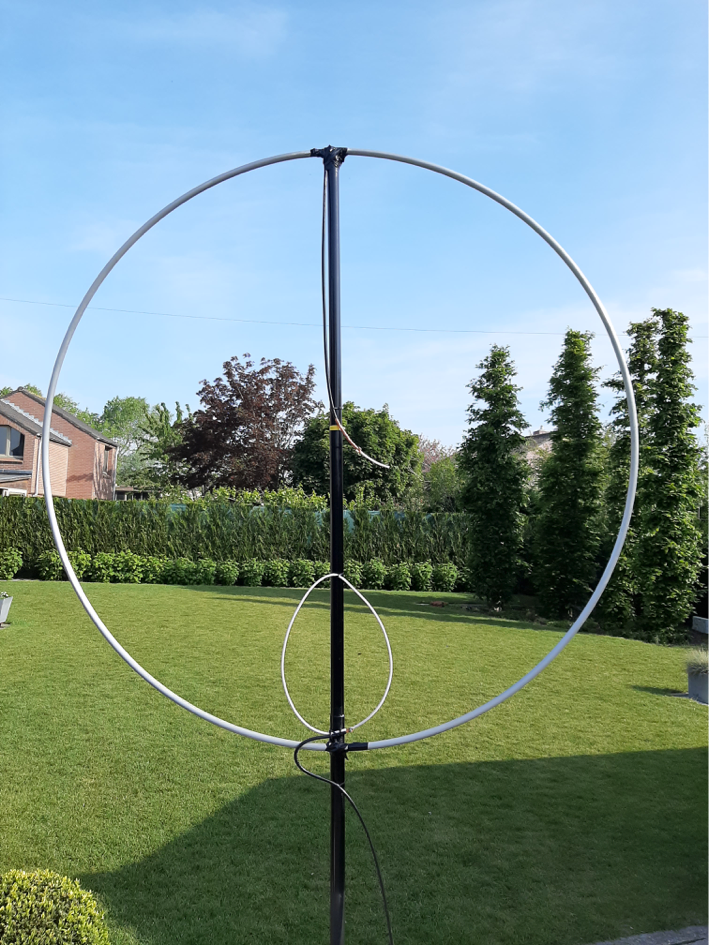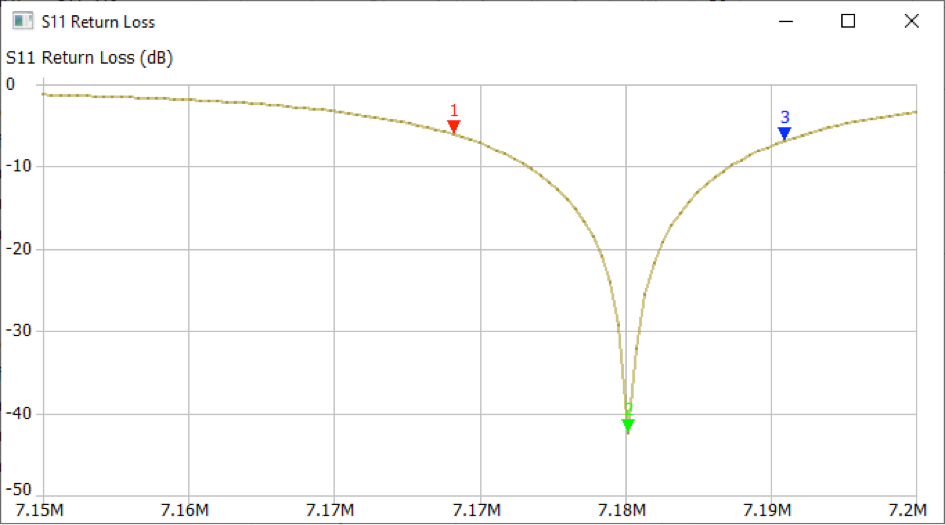top of page
Search
• xtgaby

# Magnetic loop 40 meter band.

Updated: May 30, 2020Alu tube 22 mm diameter lengt = 6 meter ==> diameter loop = 2 meter.

Small loop to insert the signal =1.30 meter for best SWR figures.At the top of the loop i have placed a meter coax cable Ecoflex 10 to create the capacitance (+/- 77 pF).Tuning of the resonance frequency by moving the braid of the coax cable.

Moving by 1 cm results in a frequency shift from 7.160 to 7.180 KHz

Tuning is very sensitive and sharp.By changing the signal loop closer to the large loop, the impedance wil change for best SWR.

Closer contact to the loop results in a higher impedance approx. 100 Ohm.Less coupling results in an impedance of 50 ohm. Smal effect on the resonance frequency.

Less coupling results in a lower resonance frequency of about 1 or 2 KHz.

Resonance frequency is 7.183 KHZ with a capacitor of 77pF.

Calculated coil value of the loop is 6.4 uH.

Measuring results:

Marker 1 is SWR = 3 lower frequency,

Marker 2 is SWR =1,

Marker 3 is SWR = 3 higher frequency.This means that we have an SWR =3 bandwith of 7.192,5 – 7.173,5 = 19 KHz.

SWR curve:Return loss curve: